# Lecture 3 newton_laws

17 de Nov de 2014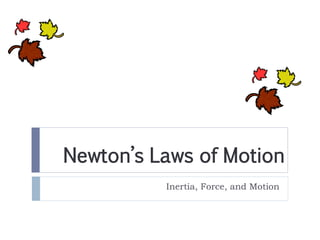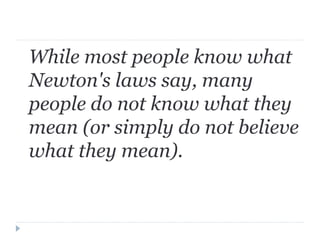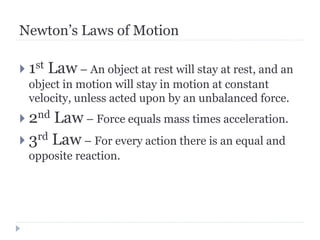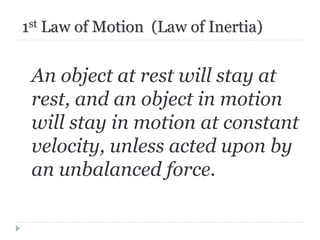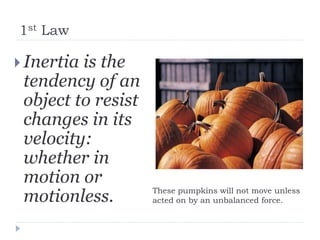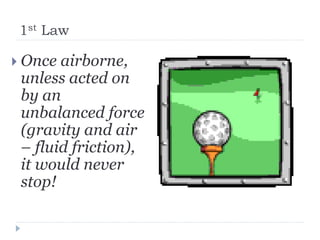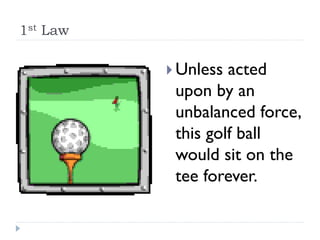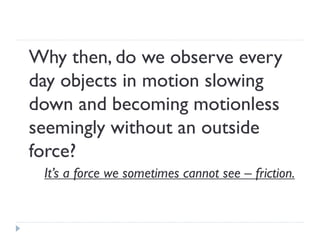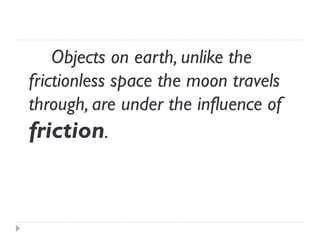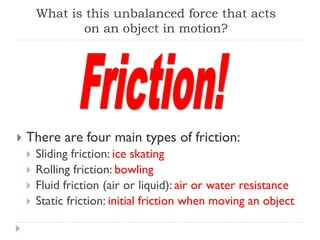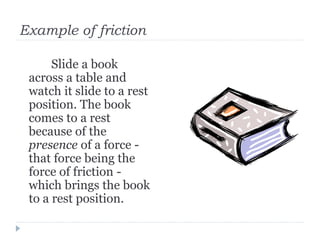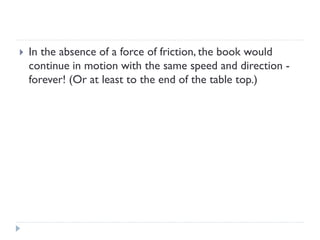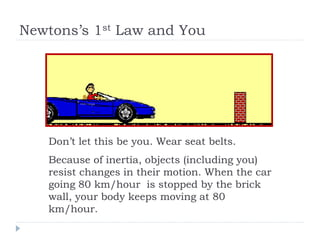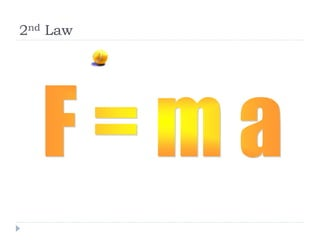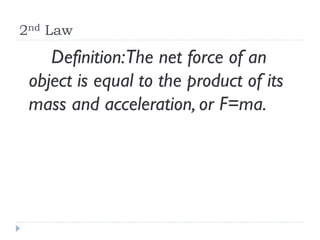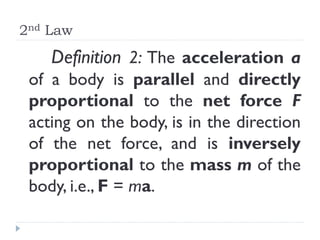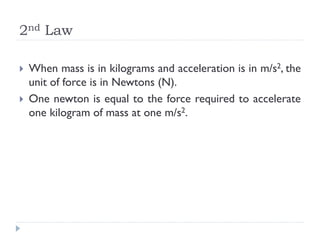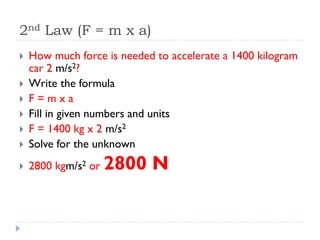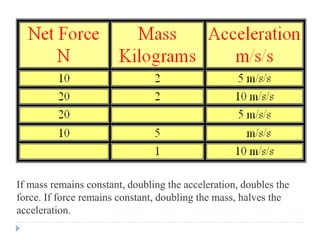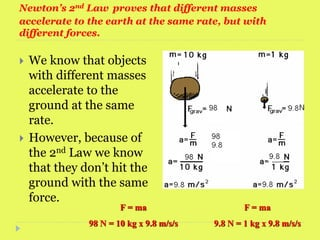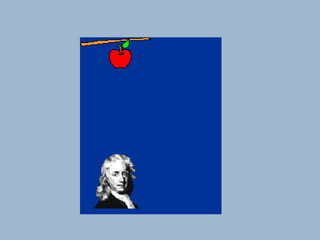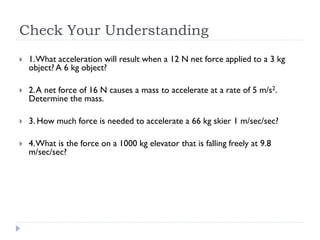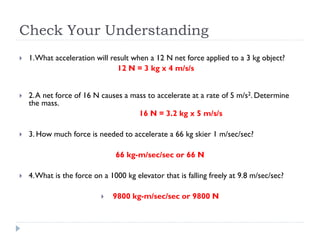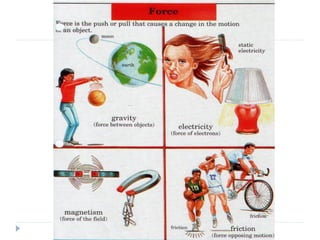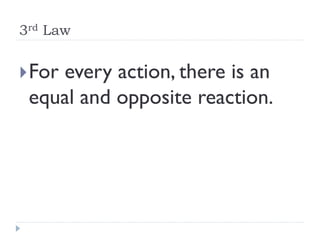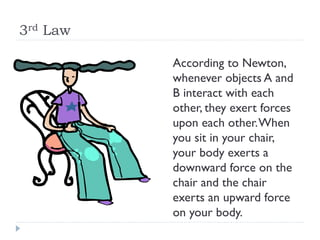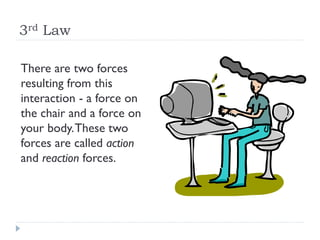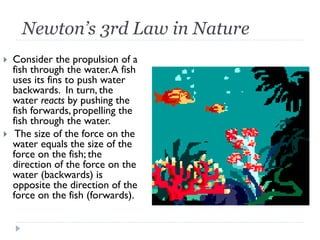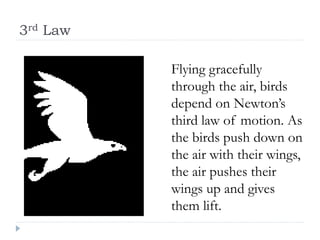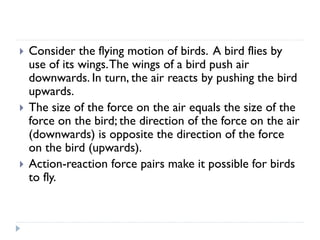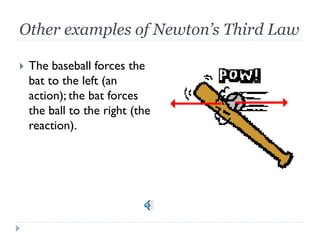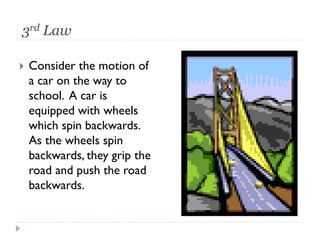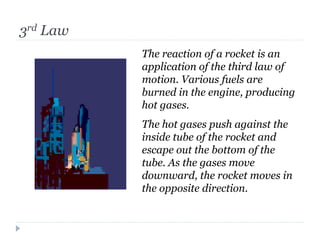1 de 34

### Lecture 3 newton_laws

• 1. Newton’s Laws of Motion Inertia, Force, and Motion
• 2. While most people know what Newton's laws say, many people do not know what they mean (or simply do not believe what they mean).
• 3. Newton’s Laws of Motion 1st Law – An object at rest will stay at rest, and an object in motion will stay in motion at constant velocity, unless acted upon by an unbalanced force. 2nd Law – Force equals mass times acceleration. 3rd Law – For every action there is an equal and opposite reaction.
• 4. 1st Law of Motion (Law of Inertia) An object at rest will stay at rest, and an object in motion will stay in motion at constant velocity, unless acted upon by an unbalanced force.
• 5. 1st Law Inertia is the tendency of an object to resist changes in its velocity: whether in motion or motionless. These pumpkins will not move unless acted on by an unbalanced force.
• 6. 1st Law Once airborne, unless acted on by an unbalanced force (gravity and air – fluid friction), it would never stop!
• 7. 1st Law Unless acted upon by an unbalanced force, this golf ball would sit on the tee forever.
• 8. Why then, do we observe every day objects in motion slowing down and becoming motionless seemingly without an outside force? It’s a force we sometimes cannot see – friction.
• 9. Objects on earth, unlike the frictionless space the moon travels through, are under the influence of friction.
• 10. There are four main types of friction: Sliding friction: ice skating Rolling friction: bowling Fluid friction (air or liquid): air or water resistance Static friction: initial friction when moving an object What is this unbalanced force that acts on an object in motion?
• 11. Example of friction Slide a book across a table and watch it slide to a rest position. The book comes to a rest because of the presence of a force - that force being the force of friction - which brings the book to a rest position.
• 12. In the absence of a force of friction, the book would continue in motion with the same speed and direction - forever! (Or at least to the end of the table top.)
• 13. Newtons’s 1st Law and You Don’t let this be you. Wear seat belts. Because of inertia, objects (including you) resist changes in their motion. When the car going 80 km/hour is stopped by the brick wall, your body keeps moving at 80 km/hour.
• 15. 2nd Law Definition: The net force of an object is equal to the product of its mass and acceleration, or F=ma.
• 16. 2nd Law Definition 2: The acceleration a of a body is parallel and directly proportional to the net force F acting on the body, is in the direction of the net force, and is inversely proportional to the mass m of the body, i.e., F = ma.
• 17. 2nd Law When mass is in kilograms and acceleration is in m/s2, the unit of force is in Newtons (N). One newton is equal to the force required to accelerate one kilogram of mass at one m/s2.
• 18. 2nd Law (F = m x a) How much force is needed to accelerate a 1400 kilogram car 2 m/s2? Write the formula F = m x a Fill in given numbers and units F = 1400 kg x 2 m/s2 Solve for the unknown 2800 kgm/s2 or 2800 N
• 19. If mass remains constant, doubling the acceleration, doubles the force. If force remains constant, doubling the mass, halves the acceleration.
• 20. Newton’s 2nd Law proves that different masses accelerate to the earth at the same rate, but with different forces. We know that objects with different masses accelerate to the ground at the same rate. However, because of the 2nd Law we know that they don’t hit the ground with the same force. F = ma 98 N = 10 kg x 9.8 m/s/s F = ma 9.8 N = 1 kg x 9.8 m/s/s
• 22. Check Your Understanding 1. What acceleration will result when a 12 N net force applied to a 3 kg object? A 6 kg object? 2. A net force of 16 N causes a mass to accelerate at a rate of 5 m/s2. Determine the mass. 3. How much force is needed to accelerate a 66 kg skier 1 m/sec/sec? 4. What is the force on a 1000 kg elevator that is falling freely at 9.8 m/sec/sec?
• 23. Check Your Understanding 1. What acceleration will result when a 12 N net force applied to a 3 kg object? 12 N = 3 kg x 4 m/s/s 2. A net force of 16 N causes a mass to accelerate at a rate of 5 m/s2. Determine the mass. 16 N = 3.2 kg x 5 m/s/s 3. How much force is needed to accelerate a 66 kg skier 1 m/sec/sec? 66 kg-m/sec/sec or 66 N 4. What is the force on a 1000 kg elevator that is falling freely at 9.8 m/sec/sec?  9800 kg-m/sec/sec or 9800 N
• 25. 3rd Law For every action, there is an equal and opposite reaction.
• 26. 3rd Law According to Newton, whenever objects A and B interact with each other, they exert forces upon each other. When you sit in your chair, your body exerts a downward force on the chair and the chair exerts an upward force on your body.
• 27. 3rd Law There are two forces resulting from this interaction - a force on the chair and a force on your body. These two forces are called action and reaction forces.
• 28. Newton’s 3rd Law in Nature Consider the propulsion of a fish through the water. A fish uses its fins to push water backwards. In turn, the water reacts by pushing the fish forwards, propelling the fish through the water.  The size of the force on the water equals the size of the force on the fish; the direction of the force on the water (backwards) is opposite the direction of the force on the fish (forwards).
• 29. 3rd Law Flying gracefully through the air, birds depend on Newton’s third law of motion. As the birds push down on the air with their wings, the air pushes their wings up and gives them lift.
• 30. Consider the flying motion of birds. A bird flies by use of its wings. The wings of a bird push air downwards. In turn, the air reacts by pushing the bird upwards. The size of the force on the air equals the size of the force on the bird; the direction of the force on the air (downwards) is opposite the direction of the force on the bird (upwards). Action-reaction force pairs make it possible for birds to fly.
• 32. Other examples of Newton’s Third Law The baseball forces the bat to the left (an action); the bat forces the ball to the right (the reaction).
• 33. 3rd Law Consider the motion of a car on the way to school. A car is equipped with wheels which spin backwards. As the wheels spin backwards, they grip the road and push the road backwards.
• 34. 3rd Law The reaction of a rocket is an application of the third law of motion. Various fuels are burned in the engine, producing hot gases. The hot gases push against the inside tube of the rocket and escape out the bottom of the tube. As the gases move downward, the rocket moves in the opposite direction.